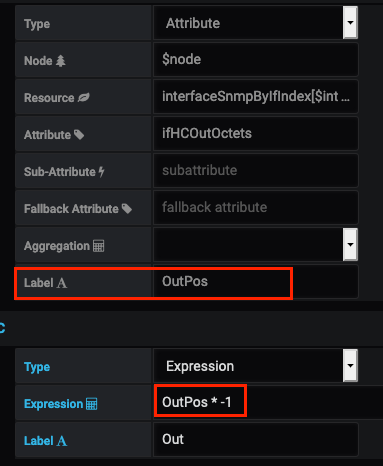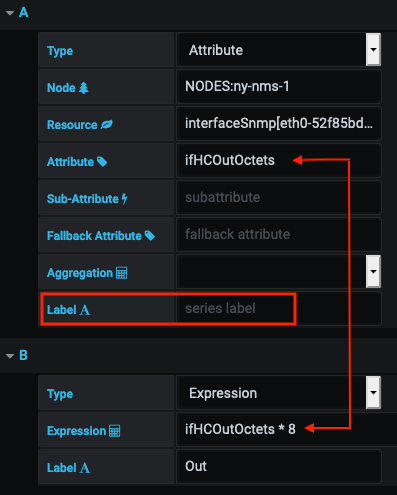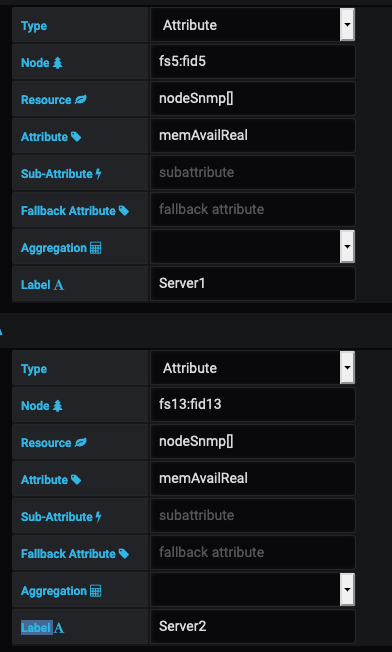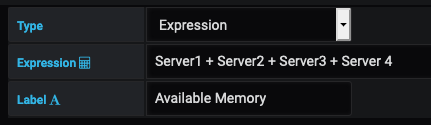# Expressions

The OpenNMS Plugin for Grafana supports JEXL mathematical and conditional operators in performance data queries. You can use them to combine or otherwise transform one or more series into a new series. For example, you can specify a filter to display the sum of data from different attribute queries (such as available memory on two nodes).

This section describes how to use expressions in queries. For more information on expressions, see the following documentation:

## Create an expression

Before you can create an expression, you must define one or more attribute queries on a Performance datasource. You can then use the attribute name or the series label (attribute alias) as part of the expression.

### Make traffic out values negative

This example queries on the total number of octets transmitted out of an interface, with an expression that changes the value to a negative number:Because traffic in and out values might be similar, and therefore would overlap on a graph, setting the `out` value to a negative number will mitigate overlap. This makes your visualizations easier to read.

 The expression uses the `OutPos` series label as the alias for `IfHCOutOctets`.

### Get byte value in bits

For this example, the `ifHCOutOctets` value is presented in bytes. If you want to display it in bits, you can create a simple expression to multiply the data by eight: `ifHCOutOctets * 8`. Note that in this case there is no series label, so the expression uses the attribute’s name:For this example, imagine that you are running a distributed cache across multiple servers. You might want to determine the amount of memory that is available across all of the servers. To do so, first create attribute queries on `memAvailReal` (the amount of physical memory currently available) for each server:Next, create an expression query that adds each series together:### Add a series with null value expression

In situations where a returned value is not a number (`NA`), using a `null` value will ensure that your expression returns a number. For example, if you are adding `SeriesA + SeriesB`, and `SeriesB` is `NA`, but `SeriesA` is a number (for example, 5), the expression becomes `5 + NA`, which equals `NA`. An expression that treats `NA` as a `null` value means that the expression would become `5 + 0`, which provides a numeric answer:

``(A == null ? 0 : A) + (B == null ? 0 : B)``
 JEXL implements an abbreviation for non-`null`, non-`false` ternary: `(A ?: 0) + (B ?: 0)`.